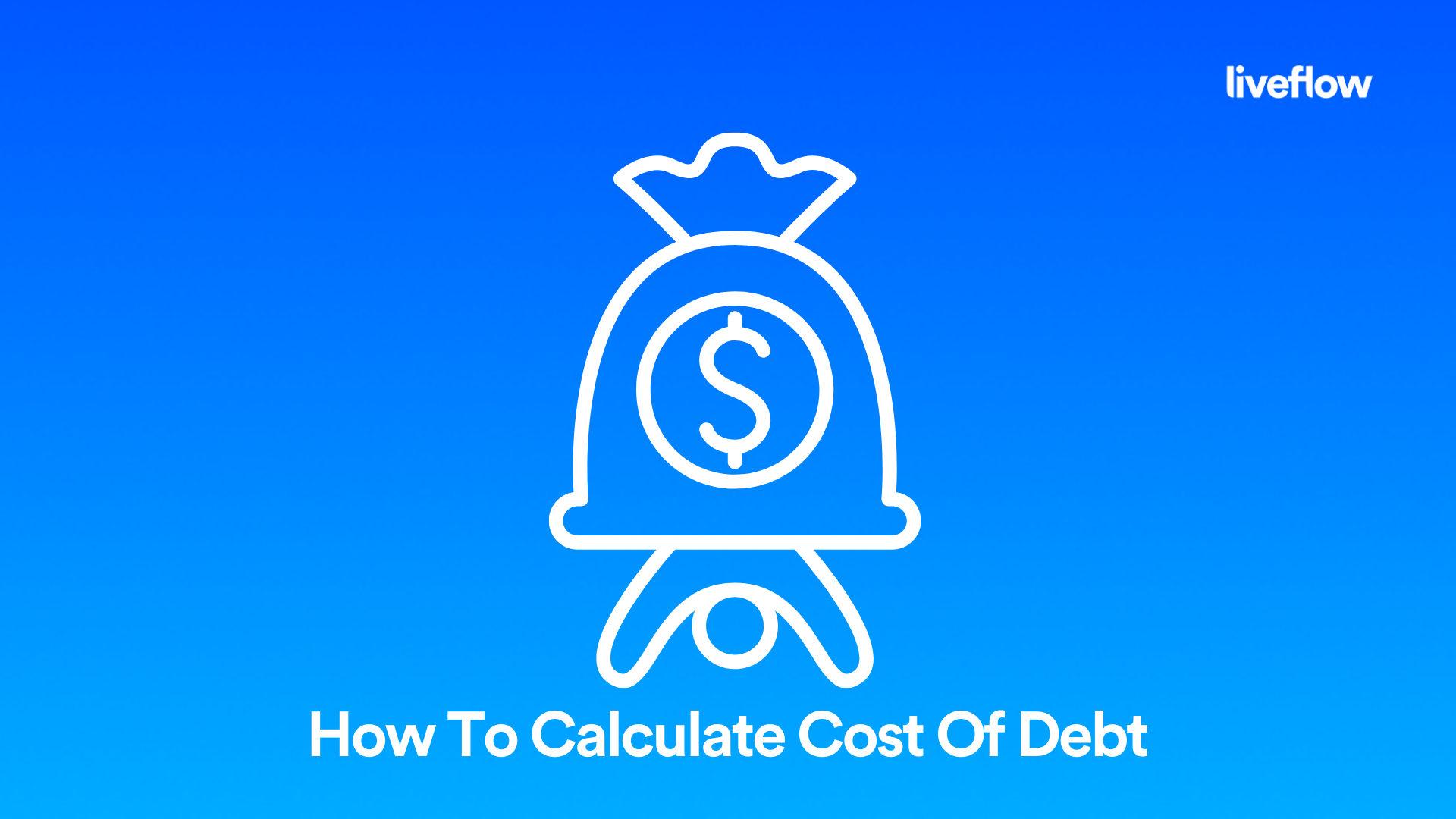# How To Calculate Cost Of Debt

July 20, 2022If you own a business or if you’re thinking about starting a business, then you may have heard the term “cost of debt formula.” But what exactly is cost of debt? Why is it important? And how is it calculated? This article will answer those questions and give some examples so that you understand this important financial principle.

## What is Cost of Debt?

Cost of debt is a financial ratio used to measure the amount of money a company pays in interest on its outstanding debt. This number helps companies figure out how much money they need to pay in interest on loans, credit facilities, and other debts, which is an important consideration when determining profitability.

## What is Cost of Debt Ratio?

The cost of debt ratio is essentially the percentage of money a company owes in interest and other charges for the money it borrows from lenders in order to operate. By determining this ratio, you can get an idea of how much the company owes compared to it’s assets.

## How Do You Calculate Cost of Debt?

To calculate the cost of debt, you need to know how much money you've borrowed from others and what rate you're being charged for that loan or credit facility. You also need to know the term of your loan so that you can calculate the total amount you will pay back by the time the loan is settled. You can figure this out by using the cost of debt formula, which is discussed below.

## The Cost of Debt Formula

There are two main components to determining your cost of debt: the interest rate and the term or length of the loan. The interest rate is just that, the rate at which your lender charges you for borrowing their money. The term is how long you have until you need to pay back what you borrowed. Below we look at the after tax cost of debt formula so you can calculate accurately using the

## How Do You Calculate KD Cost of Debt?

Calculating the cost of debt formula for cost of debt: If you’re wondering how to find cost of debt, you can use this excel pretax cost of debt formula.

Cost of Debt = (Interest Rate) x (Term)

So, let's say you want to borrow \$10,000 from your bank for one year at an interest rate of 4%. You can plug those numbers into the formula like this:

Cost of Debt = (\$10,000 x 0.04) = \$400

This means that over the course of one year, your loan will cost you \$400 in interest payments alone! To find out how much more than \$400 it will actually be, you need to know how much time is left in this loan term. That's where "term" comes in; it refers to how long you have until the loan is paid off entirely.

Once you understand the simple cost of debt formula above, it is easy to add in the term figure to determine your total cost of debt over the life of that loan. So, using the same example, if you wanted to borrow \$10,000 for three years instead of one year, you would simply multiply the cost of debt by a factor of three, like this:

Cost of Debt = (\$10,000 x 0.04 x 3) = \$1200

By using these formulas, you can calculate the cost of debt with a high degree of accuracy. However, these formulas will only work for simple debt, such as a loan with a fixed interest rate and fixed term or a credit facility with a fixed interest rate.

## Cost of Debt Example

Let's say you run a small business, and you need to borrow \$10,000 to buy new equipment. You're going to use the equipment in your business to make more money, so you can pay back the loan with interest.

You go to a bank for a loan, and they agree to give you \$10,000. They charge 5% interest per year on that loan, so at the end of one year, they will have charged you \$500 in interest (5% of \$10,000). In this example, your cost of debt is \$500.

## How to Reduce Cost of Debt

There are a few different ways that a business can reduce its cost of debt and decrease the amount of money they need to repay on their loans and other debts:

1. They can try to reduce their interest rate. This will lower their overall debt costs and make it easier for them to pay off their loans.

2. They can try to negotiate better terms with their creditors. This could include extending loan repayment periods or allowing them to pay less than the full amount due each month.

3. They could consider refinancing their current debts by taking out new loans with lower interest rates and more favorable terms.

## Summary

When you operate a business, chances are you will have some debt. The debt may be short-term or long-term, but it is simply part of running a business. It doesn't have to be a negative thing, as many businesses can manage this debt and come out of it in better shape for the future.

But, in order to stay profitable in the long run, you need to know how much that debt is costing you, which is known as the cost of debt. You can calculate the cost of debt by using the cost of debt formula above. And remember, if your cost of debt is too high, you can always try renegotiating or refinancing.

## Recommendation

If you want to understand your business finances in more detail so that you can gain actionable insights from your financial data, then consider using LiveFlow. As one of the best business finance tools on the market, LiveFlow can empower you to see the big picture and take control of your company's financial future.